# Transformer and BERT

Aug. 6, 2020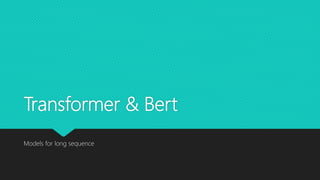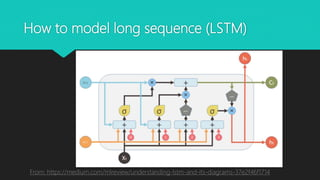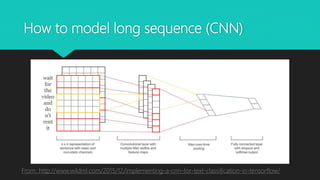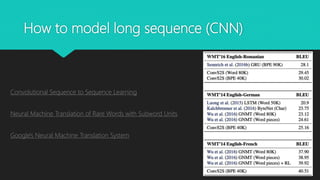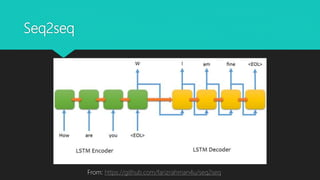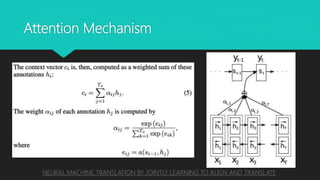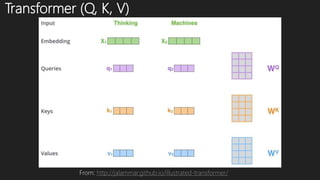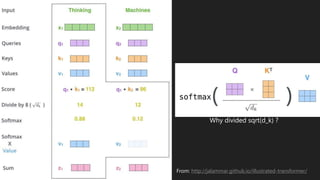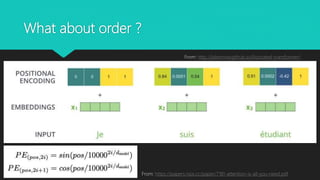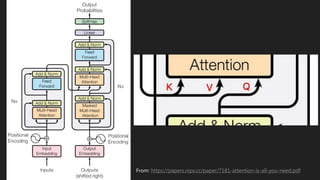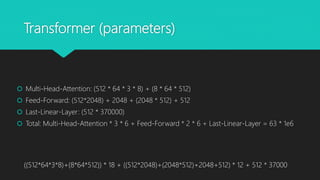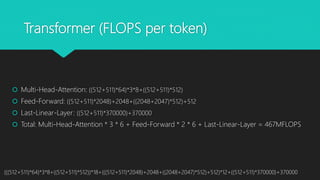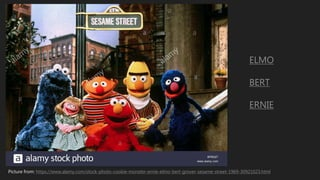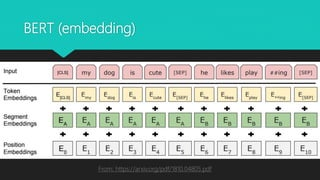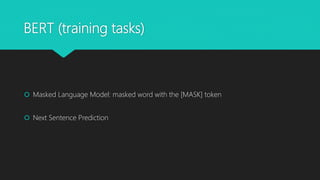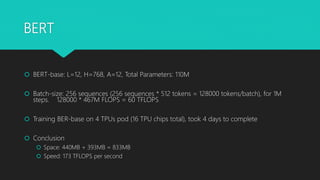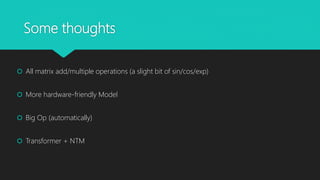1 of 19

### Transformer and BERT

1. Transformer & Bert Models for long sequence
2. How to model long sequence (LSTM) From: https://medium.com/mlreview/understanding-lstm-and-its-diagrams-37e2f46f1714
3. How to model long sequence (CNN) From: http://www.wildml.com/2015/12/implementing-a-cnn-for-text-classification-in-tensorflow/
4. How to model long sequence (CNN) Convolutional Sequence to Sequence Learning Neural Machine Translation of Rare Words with Subword Units Google's Neural Machine Translation System
5. Seq2seq From: https://github.com/farizrahman4u/seq2seq
6. Attention Mechanism NEURAL MACHINE TRANSLATION BY JOINTLY LEARNING TO ALIGN AND TRANSLATE
7. Transformer (Q, K, V) From: http://jalammar.github.io/illustrated-transformer/
8. From: http://jalammar.github.io/illustrated-transformer/ Why divided sqrt(d_k) ?
9. What about order ? From: http://jalammar.github.io/illustrated-transformer/ From: https://papers.nips.cc/paper/7181-attention-is-all-you-need.pdf
10. From: https://papers.nips.cc/paper/7181-attention-is-all-you-need.pdf
11. Transformer (parameters)  Multi-Head-Attention: (512 * 64 * 3 * 8) + (8 * 64 * 512)  Feed-Forward: (512*2048) + 2048 + (2048 * 512) + 512  Last-Linear-Layer: (512 * 370000)  Total: Multi-Head-Attention * 3 * 6 + Feed-Forward * 2 * 6 + Last-Linear-Layer = 63 * 1e6 ((512*64*3*8)+(8*64*512)) * 18 + ((512*2048)+(2048*512)+2048+512) * 12 + 512 * 37000
12. Transformer (FLOPS per token)  Multi-Head-Attention: ((512+511)*64)*3*8+((512+511)*512)  Feed-Forward: ((512+511)*2048)+2048+((2048+2047)*512)+512  Last-Linear-Layer: ((512+511)*370000)+370000  Total: Multi-Head-Attention * 3 * 6 + Feed-Forward * 2 * 6 + Last-Linear-Layer = 467MFLOPS (((512+511)*64)*3*8+((512+511)*512))*18+(((512+511)*2048)+2048+((2048+2047)*512)+512)*12+((512+511)*370000)+370000
13. Picture from: https://www.alamy.com/stock-photo-cookie-monster-ernie-elmo-bert-grover-sesame-street-1969-30921023.html ELMO BERT ERNIE
14. From: https://arxiv.org/pdf/1810.04805.pdf BERT (Origin)
15. BERT (embedding) From: https://arxiv.org/pdf/1810.04805.pdf